# Wavelet Resolution Merge

## Producer Field Guide

HGD_Product
Producer Field Guide
HGD_Portfolio_Suite
Producer

ERDAS IMAGINE Wavelet Resolution Merge allows multispectral images of relatively low spatial resolution to be sharpened using a co-registered panchromatic image of relatively higher resolution. A primary intended target dataset is Landsat 7 ETM+. Increasing the spatial resolution of multispectral imagery in this fashion is, in fact, the rationale behind the Landsat 7 sensor design.

The ERDAS IMAGINE algorithm is a modification of the work of King and Wang (King et al, 2001) with extensive input from Lemeshewsky (Lemeshewsky, 1999, Lemeshewsky, 2002a, Lemeshewsky, 2002b). Aside from traditional Pan-Multispectral image sharpening, this algorithm can be used to merge any two images, for example, radar with SPOT Pan.

Fusing information from several sensors into one composite image can take place on four levels; signal, pixel, feature, and symbolic. This algorithm works at the pixel level. The results of pixel-level fusion are primarily for presentation to a human observer/analyst (Rockinger and Fechner, 1998). However, in the case of pan/multispectral image sharpening, it must be considered that computer-based analysis (for example, supervised classification) could be a logical follow-on. Thus, it is vital that the algorithm preserve the spectral fidelity of the input dataset.

Wavelet Theory

Wavelet-based image reduction is similar to Fourier transform analysis. In the Fourier transform, long continuous (sine and cosine) waves are used as the basis. The wavelet transform uses short, discrete "wavelets" instead of a long wave. Thus the new transform is much more local (Strang et al, 1997). In image processing terms, the wavelet can be parameterized as a finite size moving window.

A key element of using wavelets is selection of the base waveform to be used; the "mother wavelet" or "basis". The "basis" is the basic waveform to be used to represent the image. The input signal (image) is broken down into successively smaller multiples of this basis.

Wavelets are derived waveforms that have a lot of mathematically useful characteristics that make them preferable to simple sine or cosine functions. For example, wavelets are discrete; that is, they have a finite length as opposed to sine waves which are continuous and infinite in length. Once the basis waveform is mathematically defined, a family of multiples can be created with incrementally increasing frequency. For example, related wavelets can be created of twice the frequency, three times the frequency, four times the frequency, and so forth.

Once the waveform family is defined, the image can be decomposed by applying coefficients to each of the waveforms. Given a sufficient number of waveforms in the family, all the detail in the image can be defined by coefficient multiples of the ever-finer waveforms.

In practice, the coefficients of the discrete high-pass filter are of more interest than the wavelets themselves. The wavelets are rarely even calculated (Shensa, 1992). In image processing, we do not want to get deeply involved in mathematical waveform decomposition; we want relatively rapid processing kernels (moving windows). Thus, we use the above theory to derive moving window, high-pass kernels which approximate the waveform decomposition.

For image processing, orthogonal and biorthogonal transforms are of interest. With orthogonal transforms, the new axes are mutually perpendicular and the output signal has the same length as the input signal. The matrices are unitary and the transform is lossless. The same filters are used for analysis and reconstruction.

In general, biorthogonal (and symmetrical) wavelets are more appropriate than orthogonal wavelets for image processing applications (Strang et al, 1997, p. 362-363). Biorthogonal wavelets are ideal for image processing applications because of their symmetry and perfect reconstruction properties. Each biorthogonal wavelet has a reconstruction order and a decomposition order associated with it. For example, biorthogonal 3.3 denotes a biorthogonal wavelet with reconstruction order 3 and decomposition order 3. For biorthogonal transforms, the lengths of and angles between the new axes may change. The new axes are not necessarily perpendicular. The analysis and reconstruction filters are not required to be the same. They are, however, mathematically constrained so that no information is lost, perfect reconstruction is possible and the matrices are invertible.

The signal processing properties of the Discrete Wavelet Transform (DWT) are strongly determined by the choice of high-pass (bandpass) filter (Shensa, 1992). Although biorthogonal wavelets are phase linear, they are shift variant due to the decimation process, which saves only even-numbered averages and differences. This means that the resultant subimage changes if the starting point is shifted (translated) by one pixel. For the commonly used, fast (Mallat, 1989) discrete wavelet decomposition algorithm, a shift of the input image can produce large changes in the values of the wavelet decomposition coefficients. One way to overcome this is to use an average of each average and difference pair.

Once selected, the wavelets are applied to the input image recursively via a pyramid algorithm or filter bank. This is commonly implemented as a cascading series of highpass and lowpass filters, based on the mother wavelet, applied sequentially to the low-pass image of the previous recursion. After filtering at any level, the low-pass image (commonly termed the approximation image) is passed to the next finer filtering in the filter bank. The high-pass images (termed horizontal, vertical, and diagonal) are retained for later image reconstruction. In practice, three or four recursions are sufficient.

2-D Discrete Wavelet Transform

A 2-D Discrete Wavelet Transform of an image yields four components:

• approximation coefficients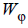• horizontal coefficients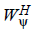– variations along the columns
• vertical coefficients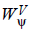– variations along the rows
• diagonal coefficients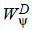– variations along the diagonals (Gonzalez and Woods, 2001)

Schematic Diagram of Discrete Wavelet Transform - DWT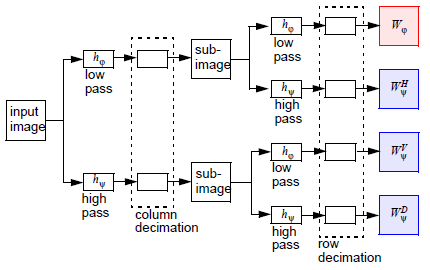Symbols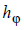and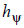are, respectively, the low-pass and high-pass wavelet filters used for decomposition. The rows of the image are convolved with the low-pass and high-pass filters and the result is downsampled along the columns. This yields two subimages whose horizontal resolutions are reduced by a factor of 2. The high-pass or detailed coefficients characterize the image’s high frequency information with vertical orientation while the low-pass component contains its low frequency, vertical information. Both subimages are again filtered columnwise with the same low-pass and high-pass filters and downsampled along rows.

Thus, for each input image, we have four subimages each reduced by a factor of 4 compared to the original image:2-D Inverse Discrete Wavelet Transform

The reduced components of the input images are passed as input to the low-pass and high-pass reconstruction filters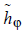and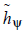(different from the ones used for decomposition) as shown in the figure below.

Inverse Discrete Wavelet Transform - DWT-1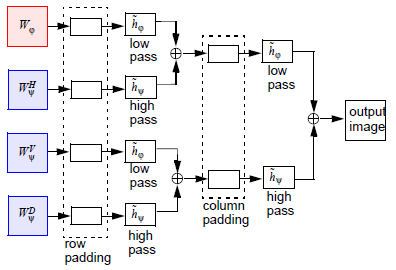The sequence of steps is the opposite of that in the DWT, the subimages are upsampled along rows (since the last step in the DWT was downsampling along rows) and convolved with the low-pass and high-pass filters columnwise (in the DWT we filtered along the columns last). These intermediate outputs are concatenated, upsampled along columns and then filtered rowwise and finally concatenated to yield the original image.

Algorithm Theory

The basic theory of the decomposition is that an image can be separated into high-frequency and low-frequency components. For example, a low-pass filter can be used to create a low-frequency image. Subtracting this low-frequency image from the original image would create the corresponding high-frequency image. These two images contain all of the information in the original image. If they were added together the result would be the original image.

The same could be done by high-pass filter filtering an image and the corresponding low-frequency image could be derived. Again, adding the two together would yield the original image. Any image can be broken into various high- and low-frequency components using various high- and low-pass filters. The wavelet family can be thought of as a high-pass filter. Thus wavelet-based high- and low-frequency images can be created from any input image. By definition, the low-frequency image is of lower resolution and the high-frequency image contains the detail of the image.

This process can be repeated recursively. The created low-frequency image could be again processed with the kernels to create new images with even lower resolution. Thus, starting with a 5-meter image, a 10-meter low-pass image and the corresponding high-pass image could be created. A second iteration would create a 20-meter low- and, corresponding, high-pass images. A third recursion would create a 40-meter low- and, corresponding, high-frequency images, and so forth.

Consider two images taken on the same day of the same area: one a 5-meter panchromatic, the other 40-meter multispectral. The 5-meter has better spatial resolution, but the 40-meter has better spectral resolution. It would be desirable to take the high-pass information from the 5-meter image and combine it with the 40-meter multispectral image yielding a 5-meter multispectral image.

Using wavelets, one can decompose the 5-meter image through several iterations until a 40-meter low-pass image is generated plus all the corresponding high-pass images derived during the recursive decomposition. This 40-meter low-pass image, derived from the original 5-meter pan image, can be replaced with the 40-meter multispectral image and the whole wavelet decomposition process reversed, using the high-pass images derived during the decomposition, to reconstruct a 5-meter resolution multispectral image. The approximation component of the high spectral resolution image and the horizontal, vertical, and diagonal components of the high spatial resolution image are fused into a new output image.

If all of the above calculations are done in a mathematically rigorously way (histomatch and resample before substitution, and so forth) one can derive a multispectral image that has the high-pass (high-frequency) details from the 5-meter image.

In the above scenario, it should be noted that the high-resolution image (panchromatic, perhaps) is a single band and so the substitution image, from the multispectral image, must also be a single band. There are tools available to compress the multispectral image into a single band for substitution using the IHS transform or PC transform. Alternately, single bands can be processed sequentially.

Wavelet Resolution Merge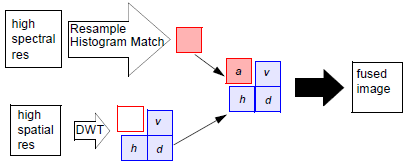Prerequisites and Limitations

Precise Coregistration

A first prerequisite is that the two images be precisely co-registered. For some sensors (for example, Landsat 7 ETM+) this co-registration is inherent in the dataset. If this is not the case, a greatly over-defined 2nd order polynomial transform should be used to coregister one image to the other. By over-defining the transform (that is, by having far more than the minimum number of tie points), it is possible to reduce the random RMS error to the subpixel level. This is easily accomplished by using the Point Prediction option in the GCP Tool. In practice, well-distributed tie points are collected until the predicted point consistently falls exactly were it should. At that time, the transform must be correct. This may require 30-60 tie points for a typical Landsat TM—SPOT Pan co-registration.

When doing the coregistration, it is generally preferable to register the lower resolution image to the higher resolution image, that is, the high resolution image is used as the Reference Image. This allows the greatest accuracy of registration. However, if the lowest resolution image has georeferencing that is to be retained, it may be desirable to use it as the Reference Image. A larger number of tie points and more attention to precise work would then be required to attain the same registration accuracy. Evaluation of X- and Y-Residual and RMS Error columns in ERDAS IMAGINE GCP CellArray indicate the accuracy of registration.

Storing the high and low resolution images as separate image files rather than Layerstacking them into a single image file is preferred. In ERDAS IMAGINE, stacked image layers are resampled to a common pixel size. Since Wavelet Resolution Merge algorithm does the pixel resampling at an optimal stage in the calculation, this avoids multiple resamplings.

After creating the coregistered images, they should be codisplayed in an ERDAS IMAGINE Viewer. Then use Fade, Flicker, and Swipe Tools to visually evaluate the precision of the coregistration.

Identical Spectral Range

Secondly, an underlying assumption of resolution merge algorithms is that the two images are spectrally identical. Thus, while a SPOT Panchromatic image can be used to sharpen TM bands 1-4, it would be questionable to use it for TM bands 5 and 7 and totally inappropriate for TM band 6 (thermal emission). If the datasets are not spectrally identical, the spectral fidelity of the MS dataset will be lost.

It has been noted (Lemeshewsky, 2002b) that there can be spectrally-induced contrast reversals between visible and NIR bands at, for example, soil-vegetation boundaries. This can produce degraded edge definition or artifacts.

Temporal Considerations

A trivial corollary is that the two images must have no temporally-induced differences. If a crop has been harvested, trees have dropped their foliage, lakes have grown or shrunk, and so forth, then merging of the two images in that area is inappropriate. If the areas of change are small, the merge can proceed and those areas removed from evaluation. If, however, the areas of change are large, the histogram matching step may introduce data distortions.

Theoretical Limitations

As described in the discussion of the discrete wavelet transform, the algorithm downsamples the high spatial resolution input image by a factor of two with each iteration. This produces approximation (a) images with pixel sizes reduced by a factor of two with each iteration. The low (spatial) resolution image will substitute exactly for the "a" image only if the input images have relative pixel sizes differing by a multiple of 2. Any other pixel size ratio will require resampling of the low (spatial) resolution image prior to substitution. Certain ratios can result in a degradation of the substitution image that may not be fully overcome by the subsequent wavelet sharpening. This will result in a less than optimal enhancement. For the most common scenarios, Landsat ETM+, IKONOS and QuickBird, this is not a problem.

Although the mathematics of the algorithm are precise for any pixel size ratio, a resolution increase of greater than two or three becomes theoretically questionable. For example, all images are degraded due to atmospheric refraction and scattering of the returning signal. This is termed "point spread". Thus, both images in a resolution merge operation have, to some (unknown) extent, been "smeared". Thus, both images in a resolution merge operation have, to an unknown extent, already been degraded. It is not reasonable to assume that each multispectral pixel can be precisely devolved into nine or more subpixels.

Spectral Transform

Three merge scenarios are possible. The simplest is when the input low (spatial) resolution image is only one band; a single band of a multispectral image, for example. In this case, the only option is to select which band to use. If the low resolution image to be processed is a multispectral image, two methods will be offered for creating the grayscale representation of the multispectral image intensity; IHS and PC.

The IHS method accepts only 3 input bands. It has been suggested that this technique produces an output image that is the best for visual interpretation. Thus, this technique would be appropriate when producing a final output product for map production. Since a visual product is likely to be only an R, G, B image, the 3-band limitation on this method is not a distinct limitation. Clearly, if one wished to sharpen more data layers, the bands could be done as separate groups of 3 and then the whole dataset layerstacked back together.

Lemeshewsky (Lemeshewsky, 2002b) discusses some theoretical limitations on IHS sharpening that suggest that sharpening of the bands individually (as discussed above) may be preferable. Yocky (Yocky, 1995) demonstrates that the IHS transform can distort colors, particularly red, and discusses theoretical explanations.

The PC Method accepts any number of input data layers. It has been suggested (Lemeshewsky, 2002a) that this technique produces an output image that better preserves the spectral integrity of the input dataset. Thus, this method would be most appropriate if further processing of the data is intended; for example, if the next step was a classification operation. Note, however, that Zhang (Zhang, 1999) has found equivocal results with the PC versus IHS approaches.

The wavelet, IHS, and PC calculations produce single precision floating point output. Consequently, the resultant image must undergo a data compression to get it back to 8-bit format.